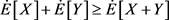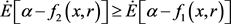﻿ 随机极限正态分布下的GCVaR风险度量研究

随机极限正态分布下的GCVaR风险度量研究 Study on Risk Measure of GCVaR under Random Limit Normal Distribution

Abstract: This paper first illustrates classical probability statistics theory and method, the incomplete applicability in the field of uncertainty financial risk measurement, and the origin of the uncertainty of the coherent risk measurement model. Then, based on the nonlinear expectation theory, by constructing the G-normal distribution of the random limit normal distribution, combining the
VaR and CVaR risk measurement model, we define the random limit risk measurement model GVaR and GCVaR. Finally, the above two risk measurement models are proved to be reasonable and appropriate coherent risk measurements.

1. 引言

2. 随机极限正态分布及其理论

$\Omega$ 为随机事件集合， $\Gamma$ 为由定义在事件集合 $\Omega$ 上的实值函数所组成的线性空间，满足：

1) $1\in \Gamma$

2) $\Gamma$ 对于局部Lipschitz函数是稳定的。即对所有的 $n\ge 1$ 和所有的 ${x}_{1},{x}_{2},\cdots ,{x}_{n}\in {C}_{l,lip}\left({R}^{n}\right)$，则同样有 $\phi \left({x}_{1},\cdots ,{x}_{n}\right)\in \Gamma$ 。其中 ${C}_{l,lip}\left({R}^{n}\right)$ 表示所有 $\phi$ 的线性空间， $\phi$ 满足：

$|\phi \left(x\right)-\phi \left(y\right)|\le C\left(1+{|x|}^{k}+{|y|}^{k}\right)|x-y|,\forall x,y\in {R}^{n}$

$C>0,k\in N$ 仅依赖于 $\phi$ 。集合 $\Gamma$ 可以理解为随机事件集合 $\Omega$ 上的随机变量空间。

$\stackrel{˙}{E}$ 为一个次线性期望，即G-期望，则 $\stackrel{˙}{E}:\Gamma \to R$ 满足下列条件：

1) 单调性： $\forall X,Y\in \Gamma$，如果 $X\ge Y$，则 $\stackrel{˙}{E}\left[X\right]\ge \stackrel{˙}{E}\left[Y\right]$

2) 保常性： $c\in R$，则 $\stackrel{˙}{E}\left[c\right]=c$

3) 次可加性： $\forall X,Y\in \Gamma$，则4) 正齐性： $\forall \lambda \ge 0,X\in \Gamma ,\stackrel{˙}{E}\left[\lambda X\right]=\lambda \stackrel{˙}{E}\left[X\right]$

$\stackrel{˙}{E}$ 满足以上4条件时， $\left(\Omega ,\Gamma ,\stackrel{˙}{E}\right)$ 为次线性期望空间。它是对一般概率空间 $\left(\Omega ,F,P\right)$ 上线性期望 $E\left(X\right)={\int }_{\Omega }X\text{d}P,X\in \left(\Omega ,F,P\right)$ 的扩展。而若仅满足条件(a)，(b)，则称 $\stackrel{˙}{E}$ 为非线性期望， $\left(\Omega ,\Gamma ,\stackrel{˙}{E}\right)$ 为非线性期望空间。

$\stackrel{˙}{E}\left[X\right]=-\stackrel{˙}{E}\left[-X\right]=0,{\stackrel{¯}{\sigma }}^{2}=\stackrel{˙}{E}\left[{X}^{2}\right],{\underset{_}{\rho }}^{2}=-\stackrel{˙}{E}\left[-{X}^{2}\right]$

1) 当 $\phi$ 为凸函数，即

$\phi \left(\lambda x+\left(1-\lambda \right)y\right)\le \lambda \phi \left(x\right)+\left(1-\lambda \right)\phi \left(y\right),\forall x,y\in R,\lambda \in \left[0,1\right]$

$\stackrel{˙}{E}\left[\phi \left(X\right)\right]=\frac{1}{\sqrt{2\text{π}{\stackrel{¯}{\sigma }}^{2}}}{\int }_{-\infty }^{+\infty }\phi \left(x\right)\mathrm{exp}\left(-\frac{{x}^{2}}{2{\stackrel{¯}{\sigma }}^{2}}\right)\text{d}x$

2) 当 $\phi$ 为凹函数，即

$\phi \left(\lambda x+\left(1-\lambda \right)y\right)\ge \lambda \phi \left(x\right)+\left(1-\lambda \right)\phi \left(y\right),\forall x,y\in R,\lambda \in \left[0,1\right]$

$\stackrel{˙}{E}\left[\phi \left(X\right)\right]=\frac{1}{\sqrt{2\text{π}{\underset{_}{\rho }}^{2}}}{\int }_{-\infty }^{+\infty }\phi \left(x\right)\mathrm{exp}\left(-\frac{{x}^{2}}{2{\underset{_}{\rho }}^{2}}\right)\text{d}x$

3. GVaR、GCVaR审慎风险度量

VaR (Value at Risk)方法在金融风险度量、确定内部经济资本需求、设定风险限额以及绩效评估等方面有着广泛应用，但是有关VaR的计算基本上是围绕着估计或模拟资产组合损失分布函数的某些特征而展开的。金融市场中数据往往是带有尖峰后尾的特点，它们的方差也可能不存在，有时甚至连期望都不存在，这样就无法应用VaR方法。更为遗憾的是，VaR不满足次可加性，意味着投资组合的分散化反而或许导致风险增加，这显然不合常理。

$\Theta$ 为实值随机变量X所有样本可能值的集合，它可表示为所有我们感兴趣的金融头寸所在的集合空间。那么，一个泛函 $\rho :\Theta \to R$ 是一致风险度量，对于所有 $X,Y\in \Theta$ 满足如下四条性质：

1) 单调性： $X\le Y⇒\rho \left(X\right)\le \rho \left(Y\right)$

2) 次可加性： $\rho \left(X+Y\right)\le \rho \left(X\right)+\rho \left(Y\right)$

3) 正齐性： $\rho \left(\lambda X\right)=\lambda \rho \left(X\right)$

4) 平移不变性： $\rho \left(X+a\right)=\rho \left(X\right)-a$

$r={\left({r}_{1},{r}_{2},\cdots ,{r}_{n}\right)}^{\text{T}}$ 表示投资组合中标的金融资产占投资总资产的比率， $x={\left({x}_{1},{x}_{2},\cdots ,{x}_{n}\right)}^{\text{T}}$ 表示标的金融资产投资损失率， $f\left(x,r\right)$ 表示投资组合的损失函数， $\beta$ 表示置信度，则

$Va{R}_{\beta }=\mathrm{inf}\left\{\alpha \in R:P\left(f\left(x,r\right)\le \alpha \right)\ge \beta \right\}$

$CVa{R}_{\beta }=E\left[f\left(x,r\right)|f\left(x,r\right)\ge Va{R}_{\beta }\right]=Va{R}_{\beta }+E\left[f\left(x,r\right)-Va{R}_{\beta }|f\left(x,r\right)\ge Va{R}_{\beta }\right]$

$GVa{R}_{\beta }=\mathrm{inf}\left\{\alpha \in R:\stackrel{˙}{E}\left[\alpha -f\left(x,r\right)\right]\ge \beta \right\}$

$GCVa{R}_{\beta }=\stackrel{˙}{E}\left[f\left(x,r\right)|f\left(x,r\right)\ge GVa{R}_{\beta }\right]=GVa{R}_{\beta }+\stackrel{˙}{E}\left[f\left(x,r\right)-GVa{R}_{\beta }|f\left(x,r\right)\ge GVa{R}_{\beta }\right]$

4. GVaR、GCVaR一致性风险度量

1) 单调性：对 $\forall {f}_{1}\left(x,r\right),{f}_{2}\left(x,r\right)\in \Gamma$，若 ${f}_{1}\left(x,r\right)\ge {f}_{2}\left(x,r\right)$，由非线性期望保单调性，。从而

$\begin{array}{l}GVa{R}_{\beta }\left[{f}_{1}\left(x,r\right)\right]\\ =\mathrm{inf}\left\{\alpha \in R:\stackrel{˙}{E}\left[\alpha -{f}_{1}\left(x,r\right)\right]\ge \beta \right\}\\ \le \mathrm{inf}\left\{\alpha \in R:\stackrel{˙}{E}\left[\alpha -{f}_{2}\left(x,r\right)\right]\ge \beta \right\}\\ =GVa{R}_{\beta }\left[{f}_{2}\left(x,r\right)\right]\end{array}$

2) 次可加性：对 $\forall {f}_{1}\left(x,r\right),{f}_{2}\left(x,r\right)\in \Gamma$

$\begin{array}{l}GVa{R}_{\beta }\left[{f}_{1}\left(x,r\right)+{f}_{2}\left(x,r\right)\right]\\ =\mathrm{inf}\left\{\alpha \in R:\stackrel{˙}{E}\left[\alpha -\left[{f}_{1}\left(x,r\right)+{f}_{2}\left(x,r\right)\right]\right]\ge \beta \right\}\\ =\mathrm{inf}\left\{\alpha +\gamma \in R:\stackrel{˙}{E}\left[\alpha -{f}_{1}\left(x,r\right)\right]+\stackrel{˙}{E}\left[\gamma -{f}_{2}\left(x,r\right)\right]\ge \eta \right\}\\ \le \mathrm{inf}\left\{\alpha \in R:\stackrel{˙}{E}\left[\alpha -{f}_{1}\left(x,r\right)\right]\ge \upsilon \right\}+\mathrm{inf}\left\{\gamma \in R:\stackrel{˙}{E}\left[\gamma -{f}_{2}\left(x,r\right)\right]\ge \omega \right\}\\ =GVa{R}_{\beta }\left[{f}_{1}\left(x,r\right)\right]+GVa{R}_{\beta }\left[{f}_{2}\left(x,r\right)\right]\end{array}$

3) 正齐性：对 $\forall \lambda >0$，由非线性期望的保常性和正齐性可得

$\begin{array}{l}GVa{R}_{\beta }\left[\lambda f\left(x,r\right)\right]\\ =\mathrm{inf}\left\{\alpha \in R:\stackrel{˙}{E}\left[\alpha -\lambda f\left(x,r\right)\right]\ge \beta \right\}\\ =\lambda \mathrm{inf}\left\{\upsilon \in R:\stackrel{˙}{E}\left[\upsilon -\lambda f\left(x,r\right)\right]\ge \eta \right\}\\ =\lambda GVa{R}_{\eta }\left[f\left(x,r\right)\right]\end{array}$

4) 平移不变性：对 $\forall f\left(x,r\right)\in \Gamma ,c>0$，由非线性期望的保常性得

$\begin{array}{l}GVa{R}_{\beta }\left[f\left(x,r\right)+c\right]\\ =\mathrm{inf}\left\{\alpha \in R:\stackrel{˙}{E}\left[\alpha -\left[f\left(x,r\right)+c\right]\right]\ge \beta \right\}\\ =\mathrm{inf}\left\{\alpha \in R:\stackrel{˙}{E}\left[\alpha -f\left(x,r\right)\right]\ge \beta \right\}-\mathrm{inf}\left\{\alpha \in R:\stackrel{˙}{E}\left[\alpha -c\right]\ge \beta \right\}\\ =GVa{R}_{\beta }\left[f\left(x,r\right)\right]-GVa{R}_{\beta }\left[c\right]\end{array}$

1) 单调性：对 $\forall {f}_{1}\left(x,r\right),{f}_{2}\left(x,r\right)\in \Gamma$，若 ${f}_{1}\left(x,r\right)\ge {f}_{2}\left(x,r\right)$，由GVaR为一致风险度量保单调性， $GVa{R}_{\beta }\left[{f}_{1}\left(x,r\right)\right]\le GVa{R}_{\beta }\left[{f}_{2}\left(x,r\right)\right]$ 。从而

$\begin{array}{l}GCVa{R}_{\beta }\left[{f}_{1}\left(x,r\right)\right]\\ =\stackrel{˙}{E}\left[{f}_{1}\left(x,r\right)|{f}_{1}\left(x,r\right)\ge GVa{R}_{\beta }\left[{f}_{1}\left(x,r\right)\right]\right]\\ \ge \stackrel{˙}{E}\left[{f}_{2}\left(x,r\right)|{f}_{2}\left(x,r\right)\ge GVa{R}_{\beta }\left[{f}_{2}\left(x,r\right)\right]\right]\\ =GCVa{R}_{\beta }\left[{f}_{2}\left(x,r\right)\right]\end{array}$

2) 次可加性：对 $\forall {f}_{1}\left(x,r\right),{f}_{2}\left(x,r\right)\in \Gamma$

$\begin{array}{l}GCVa{R}_{\beta }\left[{f}_{1}\left(x,r\right)+{f}_{2}\left(x,r\right)\right]\\ =\stackrel{˙}{E}\left[{f}_{1}\left(x,r\right)+{f}_{2}\left(x,r\right)|{f}_{1}+{f}_{2}\ge GVa{R}_{\beta }\left[{f}_{1}+{f}_{2}\right]\right]\\ \le \stackrel{˙}{E}\left[{f}_{1}\left(x,r\right)|{f}_{1}\ge GVa{R}_{\beta }\left[{f}_{1}\right]\right]+\stackrel{˙}{E}\left[{f}_{2}\left(x,r\right)|{f}_{2}\ge GVa{R}_{\beta }\left[{f}_{2}\right]\right]\\ =GCVa{R}_{\beta }\left[{f}_{1}\left(x,r\right)\right]+GCVa{R}_{\beta }\left[{f}_{2}\left(x,r\right)\right]\end{array}$

3) 正齐性：对 $\forall \lambda >0$，由非线性期望的保常性和正齐性可得

$\begin{array}{l}GCVa{R}_{\beta }\left[\lambda f\left(x,r\right)\right]\\ =\stackrel{˙}{E}\left[\lambda f\left(x,r\right)|\lambda f\left(x,r\right)\right]\ge GVa{R}_{\beta }\left[f\left(x,r\right)\right]\\ =\lambda \stackrel{˙}{E}\left[f\left(x,r\right)|f\left(x,r\right)\right]\ge \eta GVa{R}_{\beta }\left[f\left(x,r\right)\right]\\ =\lambda GVa{R}_{\beta }\left[f\left(x,r\right)\right]\end{array}$

4) 平移不变性：对 $\forall f\left(x,r\right)\in \Gamma ,c>0$，由非线性期望的保常性得

$\begin{array}{l}GCVa{R}_{\beta }\left[f\left(x,r\right)-c\right]\\ =\stackrel{˙}{E}\left[f\left(x,r\right)-c|f\left(x,r\right)-c\ge GVa{R}_{\beta }\left[f\left(x,r\right)\right]-c\right]\\ =\stackrel{˙}{E}\left[f\left(x,r\right)-c|f\left(x,r\right)-c\ge GVa{R}_{\beta }\left[f\left(x,r\right)\right]\right]-GVa{R}_{\beta }\left[c\right]\\ =\stackrel{˙}{E}\left[f\left(x,r\right)|f\left(x,r\right)\ge GVa{R}_{\beta }\left[f\left(x,r\right)\right]\right]-\stackrel{˙}{E}\left[c|c\ge GVa{R}_{\beta }\left[c\right]\right]\\ =GCVa{R}_{\beta }\left[f\left(x,r\right)\right]-GCVa{R}_{\beta }\left[c\right]\end{array}$

5. 结论

 宫晓琳, 杨淑振. 非线性期望理论与基于模型不确定性的风险度量[J]. 经济研究, 2015 ,11(1): 133-147.

 Peng, S.G. (2006) G-Expectation, G-Brownian Motion and Related Stochastic Calculus of Ito’s Type. Stochastic Analysis & Applications, 1, 3-25.

 Peng, S.G. (2007) G-Brownian Motion and Dynamic Risk Measure under Volatility Uncentainly. Princeton: Princeton University Press.

 王鹏. G-期望及其相关计算问题[D]: [硕士学位论文]. 上海: 上海交通大学, 2011.

 Artzner, P., Delban Eber, J.M. and Heath, D. (1999) Coherent measures of risk. Mathematical Finance, 4, 203-222.
https://doi.org/10.1111/1467-9965.00068

 Rockafellar, R.T. and Uryasev, S. (2000) Optimization of Condi-tional Value-at-Risk. Journal of Risk, 2, 493-517.
https://doi.org/10.21314/JOR.2000.038

 宋光辉, 吴超. 互联网金融风险度量模型选择研究[J]. 金融理论与实践, 2014, 12(1)：16-19.

 Morgan, J.P. (1996) Riskmetrics-Technical Document. Morgan Mearant Trust Company, New York, 133-167.

 王伟. 非线性数学期望及其在金融中的应用[D]: [博士学位论文]. 济南: 山东大学, 2006.

Top